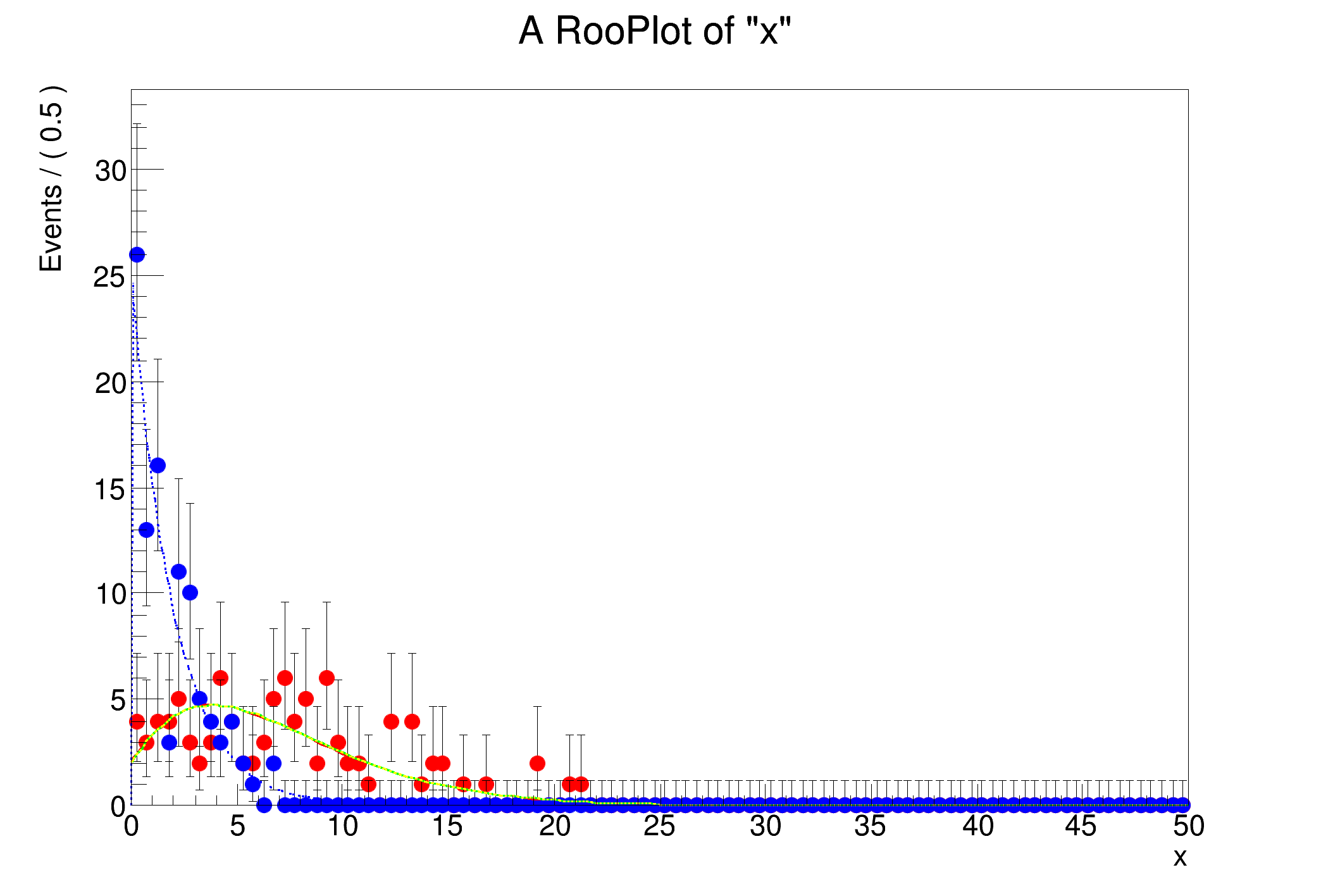ROOT   Reference GuideTestNonCentral.C File Reference

## Detailed Description␛[1mRooFit v3.60 -- Developed by Wouter Verkerke and David Kirkby␛[0m
Copyright (C) 2000-2013 NIKHEF, University of California & Stanford University
[#1] INFO:InputArguments -- RooNonCentralChiSquare sum being forced
[#0] WARNING:Eval -- RooNonCentralChiSquare did not converge: for x=36 k=2.01, lambda=5 fractional error = 0.00108172
either adjust tolerance with SetErrorTolerance(tol) or max_iter with SetMaxIter(max_it)
using namespace RooFit;
void TestNonCentral()
{
RooWorkspace w("w");
// k <2, must use sum
w.factory("NonCentralChiSquare::nc(x[0,50],k[1.99,0,5],lambda)");
// kk > 2 can use bessel
w.factory("NonCentralChiSquare::ncc(x,kk[2.01,0,5],lambda)");
// kk > 2, force sum
w.factory("NonCentralChiSquare::nccc(x,kk,lambda)");
((RooNonCentralChiSquare *)w.pdf("nccc"))->SetForceSum(true);
// a normal "central" chi-square for comparison when lambda->0
w.factory("ChiSquarePdf::cs(x,k)");
// w.var("kk")->setVal(4.); // test a large kk
RooDataSet *ncdata = w.pdf("nc")->generate(*w.var("x"), 100);
RooDataSet *csdata = w.pdf("cs")->generate(*w.var("x"), 100);
RooPlot *plot = w.var("x")->frame();
ncdata->plotOn(plot, MarkerColor(kRed));
csdata->plotOn(plot, MarkerColor(kBlue));
w.pdf("nc")->plotOn(plot, LineColor(kRed));
w.pdf("ncc")->plotOn(plot, LineColor(kGreen));
w.pdf("nccc")->plotOn(plot, LineColor(kYellow), LineStyle(kDashed));
w.pdf("cs")->plotOn(plot, LineColor(kBlue), LineStyle(kDotted));
plot->Draw();
}
@ kRed
Definition: Rtypes.h:64
@ kGreen
Definition: Rtypes.h:64
@ kBlue
Definition: Rtypes.h:64
@ kYellow
Definition: Rtypes.h:64
@ kDashed
Definition: TAttLine.h:48
@ kDotted
Definition: TAttLine.h:48
virtual RooPlot * plotOn(RooPlot *frame, const RooCmdArg &arg1=RooCmdArg::none(), const RooCmdArg &arg2=RooCmdArg::none(), const RooCmdArg &arg3=RooCmdArg::none(), const RooCmdArg &arg4=RooCmdArg::none(), const RooCmdArg &arg5=RooCmdArg::none(), const RooCmdArg &arg6=RooCmdArg::none(), const RooCmdArg &arg7=RooCmdArg::none(), const RooCmdArg &arg8=RooCmdArg::none()) const
Calls RooPlot* plotOn(RooPlot* frame, const RooLinkedList& cmdList) const ;.
Definition: RooAbsData.cxx:546
RooDataSet is a container class to hold unbinned data.
Definition: RooDataSet.h:33
The PDF of the Non-Central Chi Square distribution for n degrees of freedom.
A RooPlot is a plot frame and a container for graphics objects within that frame.
Definition: RooPlot.h:44
static RooPlot * frame(const RooAbsRealLValue &var, Double_t xmin, Double_t xmax, Int_t nBins)
Create a new frame for a given variable in x.
Definition: RooPlot.cxx:277
virtual void Draw(Option_t *options=0)
Draw this plot and all of the elements it contains.
Definition: RooPlot.cxx:712
The RooWorkspace is a persistable container for RooFit projects.
Definition: RooWorkspace.h:43
RooCmdArg MarkerColor(Color_t color)
RooCmdArg LineColor(Color_t color)
RooCmdArg LineStyle(Style_t style)
The namespace RooFit contains mostly switches that change the behaviour of functions of PDFs (or othe...

Definition in file TestNonCentral.C.